# pytorch 入门 - 优化算法详解

$w_i = w_i - \text{learning rate} * \dfrac{\partial Loss }{\partial w_i}$

• 梯度下降算法的优缺点：
缺点：
1、靠近极小值时收敛速度减慢，如下图所示；
2、直线搜索时可能会产生一些问题；
3、可能会“之字形”地下降。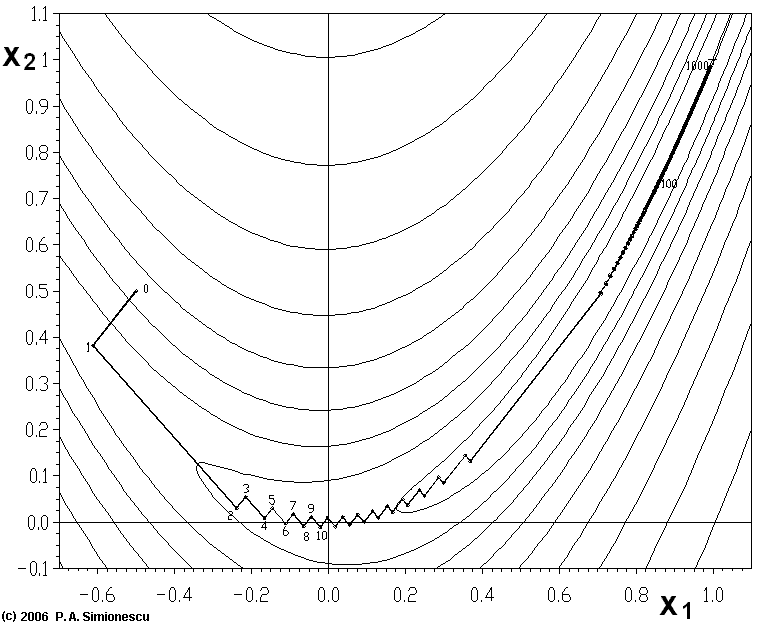从上图可以看出，梯度下降法在接近最优解的区域收敛速度明显变慢，利用梯度下降法求解需要很多次的迭代。

## GD算法的实现方式

### BGD（Batch Gradient Descent）

$W= W - lr\cdot \nabla _WJ(W)$

• BGD 缺点：计算量大，计算缓慢，对于大规模数据比较棘手；对于很大的数据集来说可能会有相似样本，这样在计算梯度过程中就容易出现冗余；不能新增样本
• BGD 优点：可以收敛到局部最优

### SGD（Stochastic Gradient Descent）

SGD在每次更新过程中，只对每个样本进行梯度更新。
$W= W - lr\cdot \nabla _WJ(W;x^{(i)};y^{(i)})$

• SGD 优点：一次只更新一个样本，计算速度很快，而且可以新增样本；SGD虽然包含了随机性，但从期望来看是等于正确导数的；稍微减小lr时，SGD与BGD收敛性一样；
• SGD 缺点：更新频繁，容易造成Loss震荡；SGD的噪音较多，使得SGD不是每次迭代都朝整体最优化方向前进；SGD收敛到局部最小存在随机性；

### MBGD （Mini-Batch Gradient Descent）

$W= W - lr\cdot \nabla _WJ(W;x^{(i:i+n)};y^{(i:i+n)})$

• MBGD 优点：降低更新参数的方差，即克服SGD噪音多不稳定的缺点，收敛更加稳定；充分利用dl学习框架中高度优化的矩阵操作进行更有效的梯度计算。

## 梯度下降算法的不足

1、不能很好保证收敛性，lr取太小的话收敛速度会过慢、lr取太大的话loss就会在极小值处不同震荡直至偏离。（措施：lr预先设置大一点，前后两次迭代的loss变化小于某个阈值后，就减小lr，但阈值的设定需要数据集本身的特点）

2、对于非凸函数，GD容易被困在局部极小值或者鞍点处（鞍点附近的loss都一样，所有维度的梯度接近0）；若是用BGD，则优化停止不动；若是MBGD或SGD，那么每次找到的梯度是不同的，会发生震荡，来回跳动

3、SGD对所有参数更新采用的lr是相同的，若数据稀疏，我们更希望对出现频率低的特征进行大一点的更新，即学习率lr是一个可变量，根据特征出现频率以及更新次数发生变化

## GD算法的改进

### Momentum

momentum是模拟物理里动量的概念，积累之前的动量来替代真正的梯度。它通过加入$\gamma v_{t-1}$来加速SGD的同时可以抑制震荡。

$v_t = \gamma v_{t-1} + lr*\nabla_w J(w)$ 一般$\gamma$常取0.9左右
$w = w-v_t$

• 缺点：缺乏先知信息，若知道快要上坡了，就知道减速的话，适应性会更好

### Nesterov Accelerated Gradient

$v_t = \gamma v_{t-1} + lr*\nabla_w J(w- \gamma v_{t-1})$ 一般$\gamma$常取0.9左右
$w = w-v_t$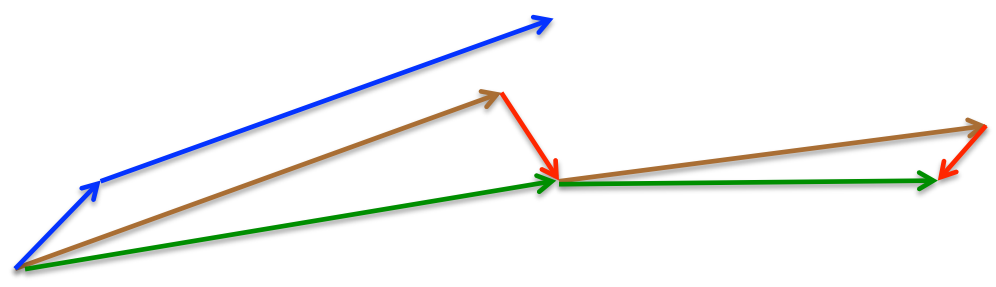momentum首先计算一个梯度(短的蓝色向量)，然后在加速更新梯度的方向进行一个大的跳跃(长的蓝色向量)，nesterov项首先在之前加速的梯度方向进行一个大的跳跃(棕色向量)，计算梯度然后进行校正(绿色梯向量)

### 自适应优化算法

$g_t = \nabla _WJ(W)$
$n_t = n_{t-1} + g_t^2$
$W_t = W_t - \dfrac{lr}{\sqrt{\smash[b]{n_t +\epsilon}}}*g_t$$\epsilon$保证分母不为0，$n_t$表示从1到t的梯度累计，形成一个约束。

1、由公式可以看出，仍依赖于人工设置一个全局学习率
2、η设置过大的话，会使regularizer过于敏感，对梯度的调节太大

$E[g^2]_t = \gamma E[g^2]_{t-1} + (1-\gamma)g_t^2$

$w = w - \dfrac{lr}{\sqrt{\smash[b]{E[g^2]_t+\epsilon}}}*g_t$#### RMSprop

RMSprop 也是为了解决Adagrad 学习率急剧下降的问题。RMSprop可以算作Adadelta的一个特例：当$\gamma$=0.5时，$E[g^2]_t = \gamma E[g^2]_{t-1} + (1-\gamma)g_t^2$就变为了求梯度平方和的平均数。 如果再求根的话，就变成了RMS(均方根)：

$w = w - \dfrac{lr}{RMS[g]_t}*g_t$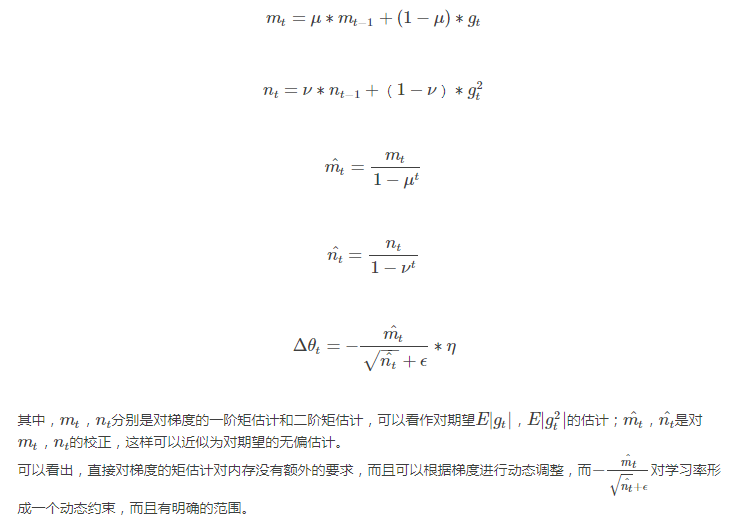Adam 就是在RMSprop 的基础上加了bias-correction 和 momentum的效果

## 优化算法的选择

SGD通常训练时间更长，容易陷入鞍点，但是在好的初始化和学习率调度方案的情况下，结果更可靠

# 牛顿法和拟牛顿法（Newton’s method & Quasi-Newton Methods）

## 基本牛顿法

（1）原理：对于一维函数问题，将函数的极值问题转化为求f’(x)=0的问题。将函数做二阶泰勒展开： 对等式两边关于x求导，得到 得到$x=x_k-\dfrac{f’(x_k)}{f’’(x_k)}$ 这是牛顿法的基本更新公式
（2）基本流程：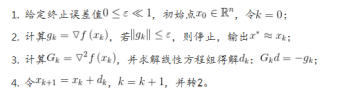1、牛顿法收敛速度很快且具有局部二阶收敛性
2、基本牛顿法依赖于初始点的选取，若初始点未足够靠近极小点，会导致算法不收敛.

## 全局牛顿法

（1）基本流程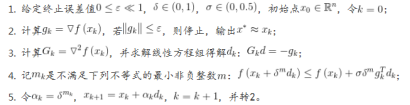（2）Armijo搜索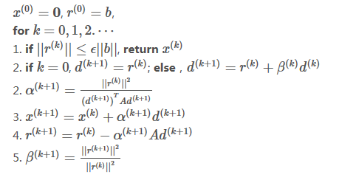# GD与另两种优化算法的比较

• 梯度下降法和牛顿法：
两者都是梯度求解，而牛顿法/拟牛顿法是用二阶海塞矩阵的逆矩阵或伪逆矩阵求解。相对而言，使用牛顿法收敛更快，但是每次迭代时间比梯度下降法长。

• 梯度下降算法和共轭梯度法：
共轭梯度法克服了梯度下降算法中的收敛慢的缺点。

# pytorch优化函数包

torch.optim是一个实现了各种优化算法的库。大部分常用的方法得到支持，并且接口具备足够的通用性，使得未来能够集成更加复杂的方法。

torch.optim.Adadelta(params, lr=1.0, rho=0.9, eps=1e-06, weight_decay=0) lr_decay (float, 可选) – 学习率衰减；weight_decay (float, 可选) – 权重衰减（L2惩罚）
torch.optim.Adam(params, lr=0.001, betas=(0.9, 0.999), eps=1e-08, weight_decay=0) betas (Tuple[float, float], 可选) – 用于计算梯度以及梯度平方的运行平均值的系数；eps (float, 可选) – 为了增加数值计算的稳定性而加到分母里的项；
torch.optim.RMSprop(params, lr=0.01, alpha=0.99, eps=1e-08, weight_decay=0, momentum=0, centered=False) momentum (float, 可选) – 动量因子；alpha (float, 可选) – 平滑常数；centered (bool, 可选) – 如果为True，计算中心化的RMSProp，并且用它的方差预测值对梯度进行归一化；
torch.optim.SGD(params, lr=, momentum=0, dampening=0, weight_decay=0, nesterov=False) dampening (float, 可选) – 动量的抑制因子；nesterov (bool, 可选) – 使用Nesterov动量
torch.optim.Adamax(params, lr=0.002, betas=(0.9, 0.999), eps=1e-08, weight_decay=0)
torch.optim.ASGD(params, lr=0.01, lambd=0.0001, alpha=0.75, t0=1000000.0,weight_decay=0) lambd (float, 可选) – 衰减项； alpha (float, 可选) – eta更新的指数 ； t0 (float, 可选) – 指明在哪一次开始平均化

optimizer = torch.optim.SGD(model.parameters(), lr=0.1, momentum=0.9)